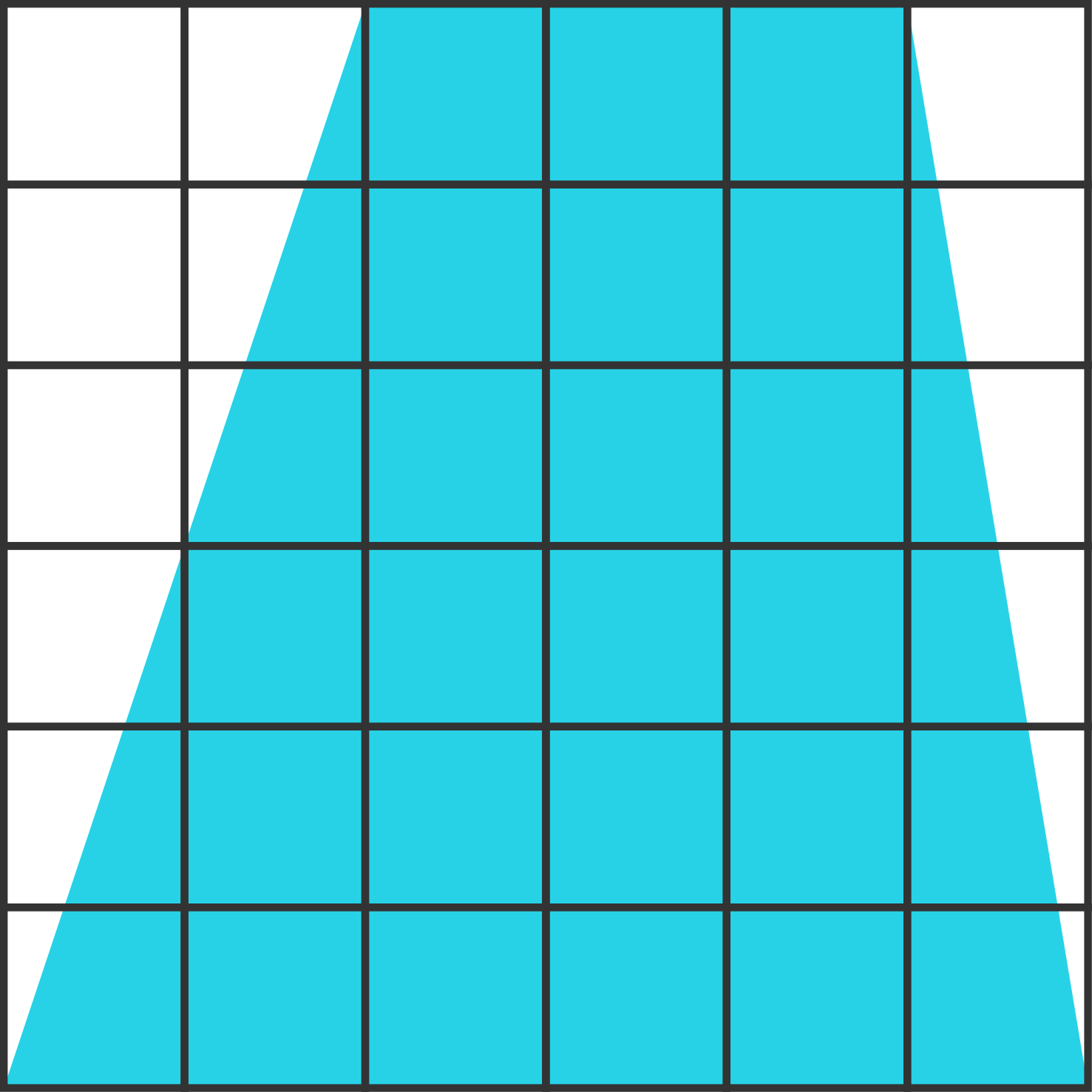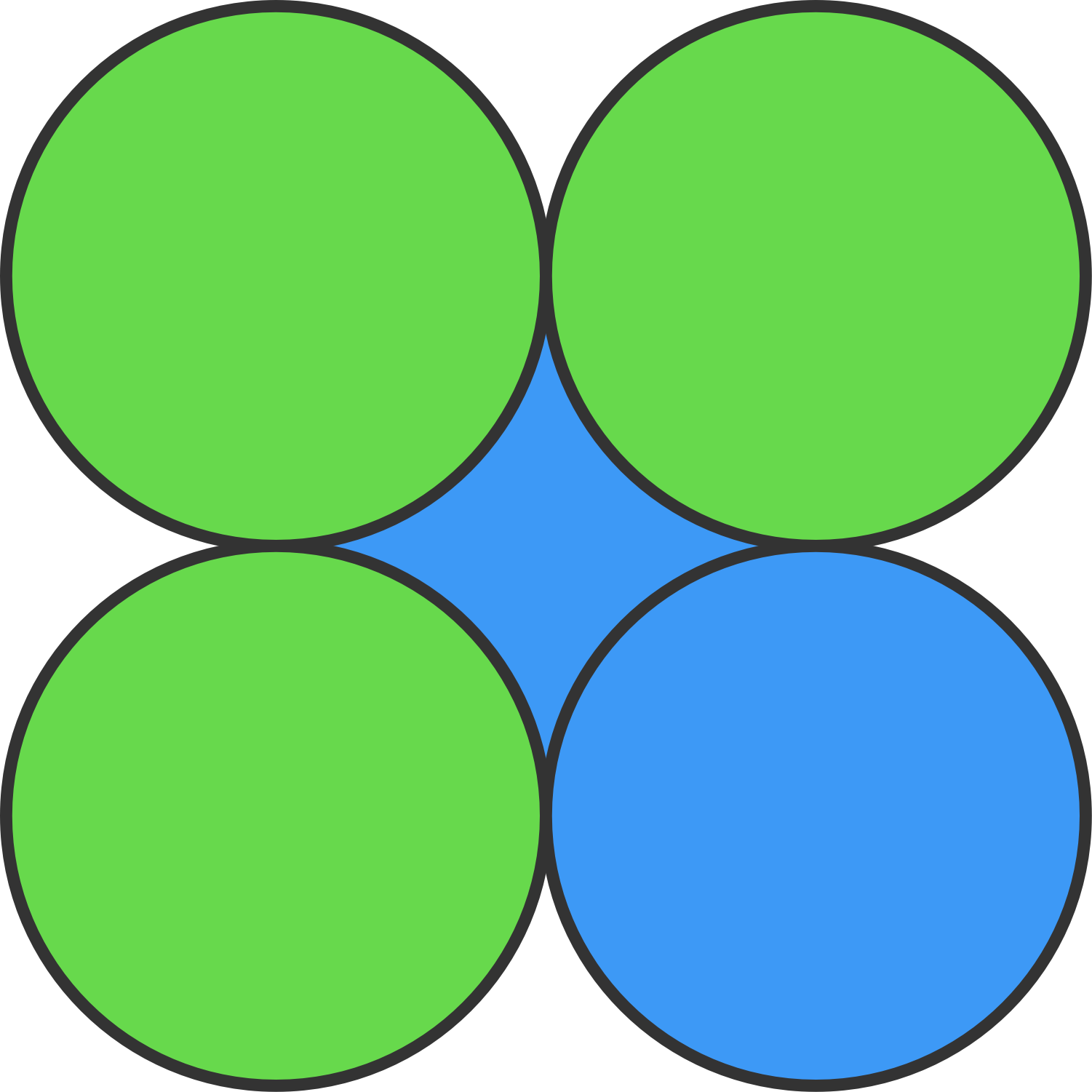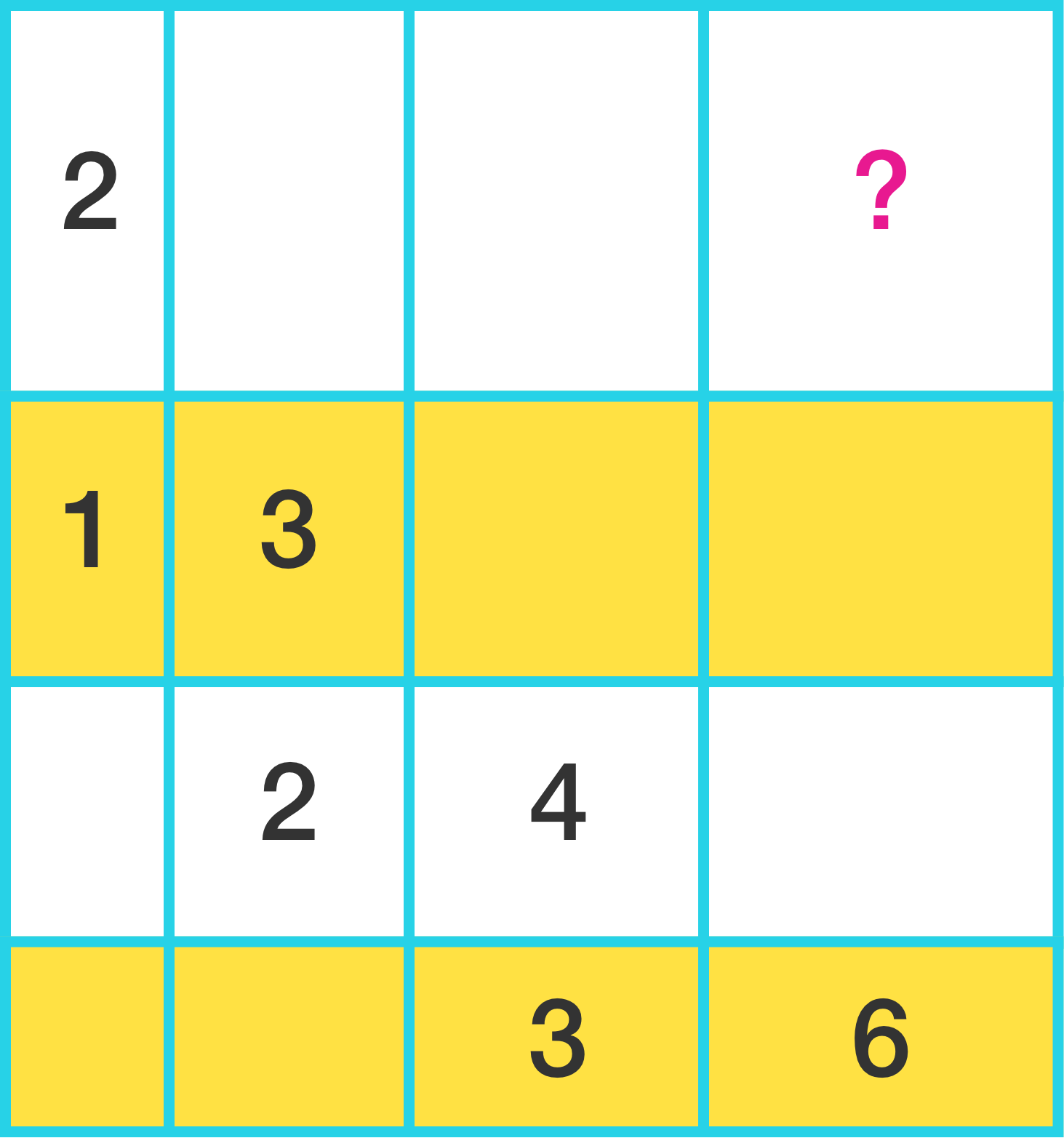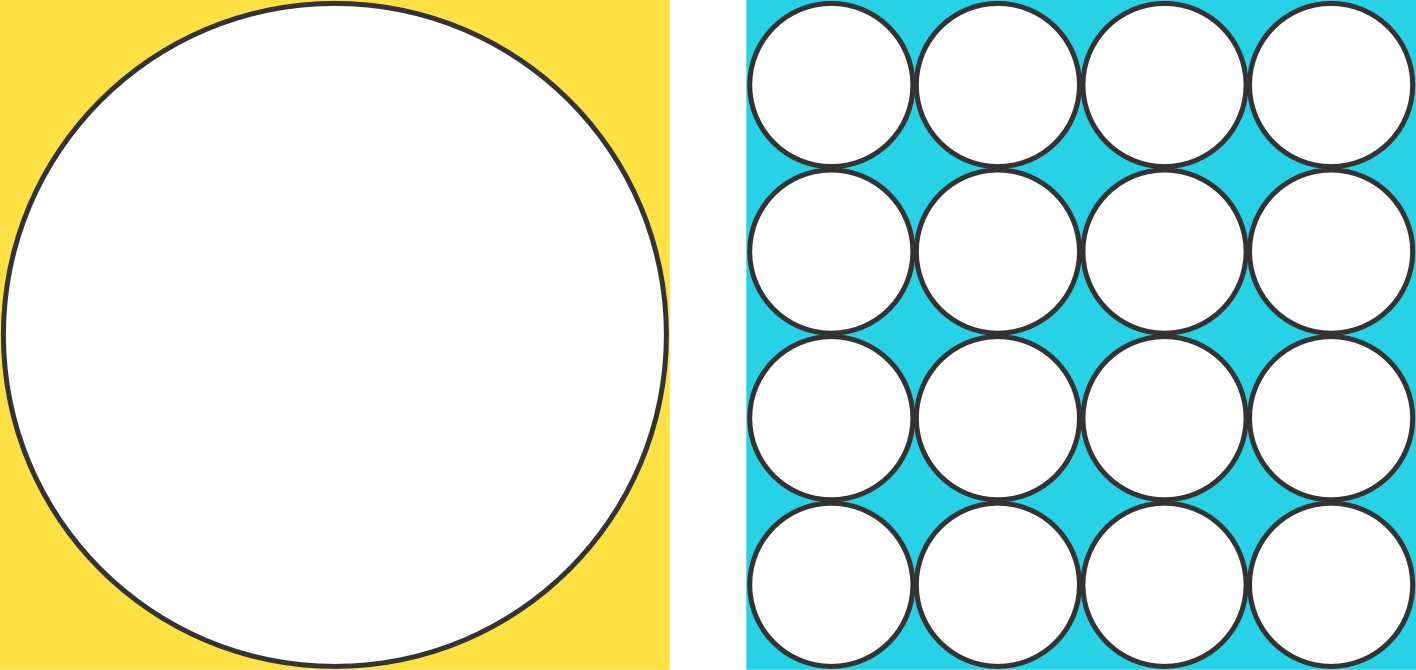Everyday Math

# Length and Area: Level 2 ChallengesIf each square has a side length of 1, what is the area of the blue trapezoid?

Note: A trapezoid has at least a pair of parallel sides. It is also known as a trapezium in the UK.

Which of the following triangles has a larger area:

• triangle A with side lengths $13, 13, 10$, or
• triangle B with side lengths $13, 13, 24\, ?$Given that all the circles have equal radius of 10 cm, what is the area of the shaded blue part?In this grid, each rectangle is labelled with its area. What area should replace the question mark?In the above figure, which shaded area is larger?

The small circles all have the same size.

×